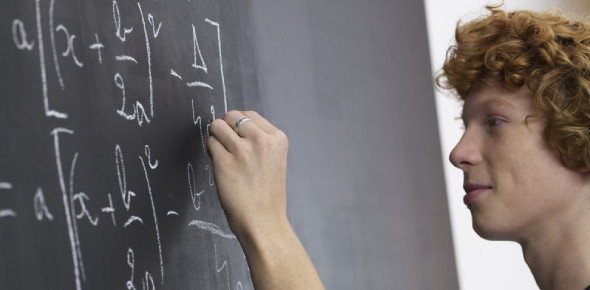# How Well Do You Know Algebra?

10 Questions | Total Attempts: 321SettingsMost people dislike math, especially algebra. However, if you must know, algebra is very important! So, how much do you think you know about algebra? Come on, test yourself with this quiz!

• 1.
Solve for x in this equation: x + 41 = 56
• A.

X = 10

• B.

X = 12

• C.

X = 15

• D.

X = 20

• 2.
3 + (-2) = y.   What is the value for y?
• A.

1

• B.

-1

• C.

2

• D.

-2

• 3.
7 + (-5) x 3 = ..
• A.

22

• B.

6

• C.

36

• D.

-8

• 4.
13 x (-4) - (-3) = ...
• A.

-52

• B.

61

• C.

-49

• D.

49

• 5.
40 : (-8) = ...
• A.

320

• B.

-5

• C.

8

• D.

5

• 6.
Solve for p. p=888+99
• A.

90

• B.

11

• C.

987

• D.

900

• 7.
What is x? x+7<8
• A.

X

• B.

1

• C.

X>1

• D.

X>2

• 8.
What's the dependent variable? X=5a
• A.

X

• B.

A

• C.

5

• D.

Not given

• 9.
A + 9 = 20
• A.

9

• B.

10

• C.

20

• D.

11

• 10.
What is the value of 'b' in the equation? b - 6 = -9
• A.

2

• B.

-3

• C.

3

• D.

-15

Related TopicsBack to top